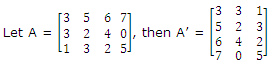# What is transpose of a matrix

Publish On: 2019-03-14

Total Post: 559

# Mjay Jollay

Total Post: 0

## ANS: What is transpose of a matrix

If A = [aij] be a matrix of order p × q then the matrix B = [bij] of order q × p is called transpose of the matrix A if bij = aij for all values of i and j, i.e. the (i, j)th element of B is equal to (j, i)th element of A.

The matrix B is also written as B = A’.

In other words, the matrix of order q × p obtained from A by interchanging its rows and columns is called transpose of A, e.g.